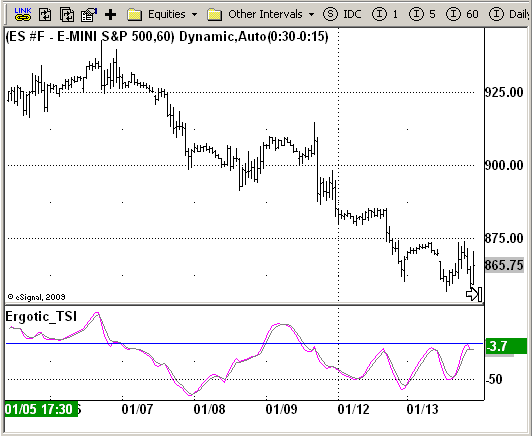# Ergotic TSI

ICE Data Services -

Ergotic_TSI.efs
EFSLibrary - Discussion Board

File Name: Ergotic_TSI.efs

Description:

Ergotic TSI

Formula Parameters:

• r - Length of first EMA smoothing of 1 day momentum : 4
• s - Length of second EMA smoothing of 1 day smoothing : 8
• u- Length of third EMA smoothing of 1 day momentum : 6
• Length of EMA signal line : 3
• Source of Ergotic TSI : Close

Notes:

This is one of the techniques described by William Blau in his book "Momentum, Direction and Divergence" (1995). If you like to learn more, we advise you to read this book. His book focuses on three key aspects of trading: momentum, direction and divergence. Blau, who was an electrical engineer before becoming a trader, thoroughly examines the relationship between price and momentum in step-by-step examples. From this grounding, he then looks at the deficiencies in other oscillators and introduces some innovative techniques, including a fresh twist on Stochastics. On directional issues, he analyzes the  intricacies of ADX and offers a unique approach to help define trending and non-trending periods.

Ergotic_TSI.efsEFS Code:

```/*********************************
Provided By:
eSignal (Copyright c eSignal), a division of Interactive Data
Formula Script (EFS) is for educational purposes only and may be
modified and saved under a new file name.  eSignal is not responsible
for the functionality once modified.  eSignal reserves the right
to modify and overwrite this EFS file with each new release.

Description:
Ergotic TSI

Version:            1.0  01/12/2009

Formula Parameters:                                         Default:
r - Length of first EMA smoothing of 1 day momentum        4
s - Length of second EMA smoothing of 1 day smoothing      8
u- Length of third EMA smoothing of 1 day momentum         6
Length of EMA signal line                                  3
Source of Ergotic TSI                                      Close

Notes:
This is one of the techniques described by William Blau in his book "Momentum,
read this book. His book focuses on three key aspects of trading: momentum,
direction and divergence. Blau, who was an electrical engineer before becoming
a trader, thoroughly examines the relationship between price and momentum in
step-by-step examples. From this grounding, he then looks at the deficiencies
in other oscillators and introduces some innovative techniques, including a
fresh twist on Stochastics. On directional issues, he analyzes the intricacies
of ADX and offers a unique approach to help define trending and non-trending periods.

**********************************/

var fpArray = new Array();
var bInit = false;

function preMain() {
setStudyTitle("Ergotic_TSI");
setCursorLabelName("ErgTSI", 0);
setCursorLabelName("SigLin", 1);
setDefaultBarFgColor(Color.fushcia, 0);
setDefaultBarFgColor(Color.grey, 1);

var x=0;
fpArray[x] = new FunctionParameter("r", FunctionParameter.NUMBER);
with(fpArray[x++]){
setLowerLimit(1);
setDefault(4);
}

fpArray[x] = new FunctionParameter("s", FunctionParameter.NUMBER);
with(fpArray[x++]){
setLowerLimit(1);
setDefault(8);
}

fpArray[x] = new FunctionParameter("u", FunctionParameter.NUMBER);
with(fpArray[x++]){
setLowerLimit(1);
setDefault(6);
}

fpArray[x] = new FunctionParameter("SmthLen", FunctionParameter.NUMBER);
with(fpArray[x++]){
setName("Length of EMA signal line");
setLowerLimit(1);
setDefault(3);
}

fpArray[x] = new FunctionParameter("sPrice", FunctionParameter.STRING);
with(fpArray[x++]){
setName("Source of Ergotic TSI");
setDefault("close");
}
}

var xPrice = null;
var xSMA_R = null;
var xSMA_aR = null;
var xPrice1 = null;
var xPrice2 = null;
var xTSI = null;
var xEMA_TSI = null;

function main(sPrice, r, s, u, SmthLen) {
var nState = getBarState();
var nTSI = 0;
var nEMA_TSI = 0;

if (nState == BARSTATE_ALLBARS) {
if (sPrice == null) sPrice = "close";
if (r == null) r = 4;
if (s == null) s = 8;
if (u == null) u = 6;
if (SmthLen == null) SmthLen = 3;
}

if(bInit == false) {
xPrice = eval(sPrice)();
xPrice1 = efsInternal("Calc_Price1", xPrice);
xPrice2 = efsInternal("Calc_Price2", xPrice);
xSMA_R = ema(u, ema(s, ema(r, xPrice1)));
xSMA_aR = ema(u, ema(s, ema(r, xPrice2)));
xTSI = efsInternal("Calc_TSI", xSMA_R, xSMA_aR);
xEMA_TSI = ema(SmthLen, xTSI);
bInit = true;
}

nTSI = xTSI.getValue(0);
nEMA_TSI = xEMA_TSI.getValue(0);

if (nEMA_TSI == null || nTSI == null) return;

return new Array(nTSI, nEMA_TSI);
}

function Calc_Price1(xPrice) {
var nRes = 0;
nRes = xPrice.getValue(0) - xPrice.getValue(-1);
if (nRes == null) nRes = 1;
return nRes;
}

function Calc_Price2(xPrice) {
var nRes = 0;
nRes = Math.abs(xPrice.getValue(0) - xPrice.getValue(-1));
if (nRes == null) nRes = 1;
return nRes;
}

function Calc_TSI(xSMA_R, xSMA_aR) {
var nRes = 0;
var Val1 = 100 * xSMA_R.getValue(0);
var Val2 = xSMA_aR.getValue(0);
if (Val2 != 0 || Val2 != null) nRes = Val1 / Val2;
if (nRes == null) return;
return nRes;
}```